# Icosahedron

## Expansion

Expansion” on polyhedra is the process of moving all faces outward from the center of polyhedron, and fill the gaps with new faces.

An expanded polyhedron has all the faces of the original polyhedron, all the faces of its dual, and new square faces in place of the original edges.

## TruncationIcosahedron with increasingly larger truncation. The outer wireframe shows its dual.

Truncation is the process of cutting the vertex.

• In truncation, when a original face becomes a regular polygon again, it's called “uniform truncation”.
• In truncation, when original edge disappears (it becomes a point), the truncation is called “complete truncation” or “rectified”.

The word “truncation” by itself without qualification usually means “uniform truncation”.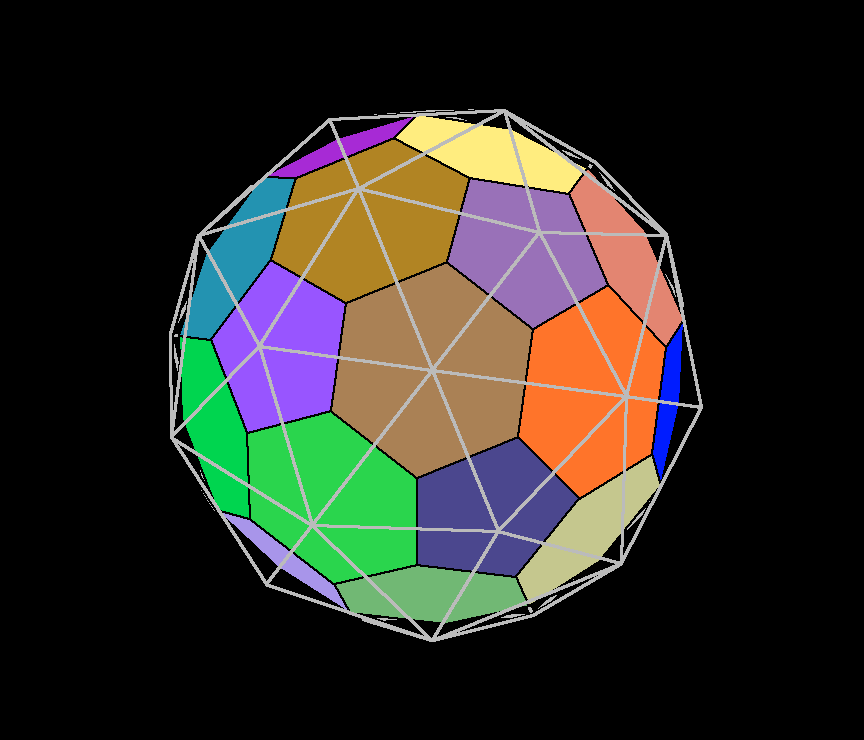“Truncated icosahedron” (aka buckyball, soccer ball pattern), and its dual “Pentakis dodecahedron” (in wireframe). “Truncated icosahedron” is the result of uniform truncation of Icosahedron. Truncated icosahedron have 12 pentagon and 20 hexagon faces.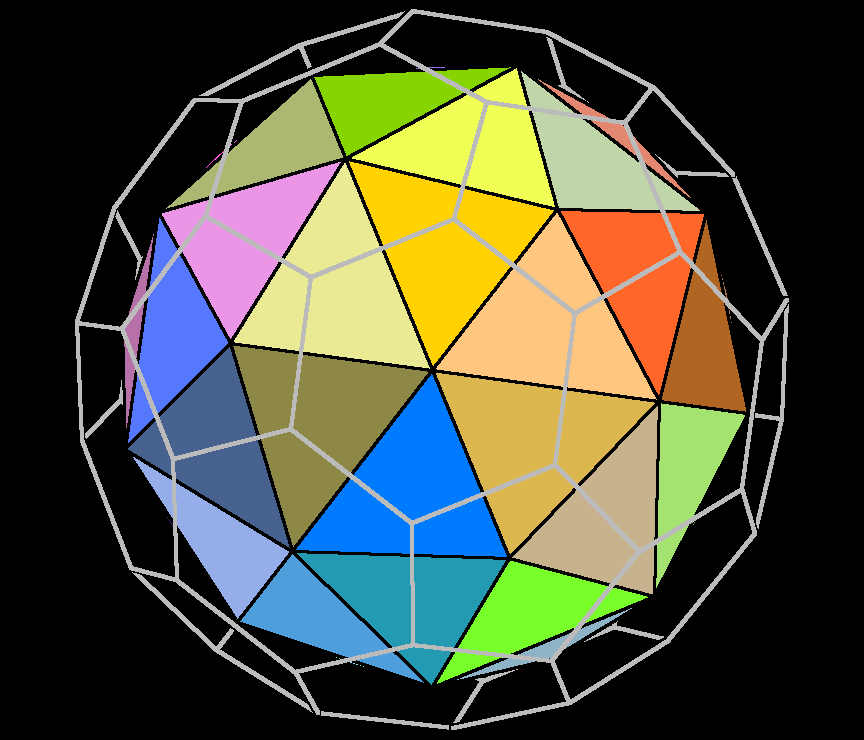“Pentakis dodecahedron”, and its dual (in wireframe) the “truncated icosahedron” (aka buckyball, soccer ball pattern). The Pentakis dodecahedron has 60 congruent triangular faces.

Dual of Truncated Icosahedron is “Pentakis dodecahedron”.

Icosahedron under rectified truncation results in “Icosidodecahedron”. Icosidodecahedron has 20 triangular faces, 12 pentagonal faces, 30 identical vertices.

Dual of Icosidodecahedron is “Rhombic triacontahedron

Both Truncated icosahedron and Icosidodecahedron are “Archimedean solids”.

## Archimedean solid

Archimedean solid” is any convex polyhedron with regular polygon faces meeting in identical vertices, excluding the 5 Platonic solids.

“Identical vertices” means that for any two vertices, there is a global isometry of the solid that takes one vertex to the other.

There are 13 Archimedean solids.

If we do not require a global symmetry that maps one vertice config to another, merely that all vertices have the same configuration, then, there are 14 Archimedean solids. The new one is called “Elongated square gyrobicupola

## Snub Truncation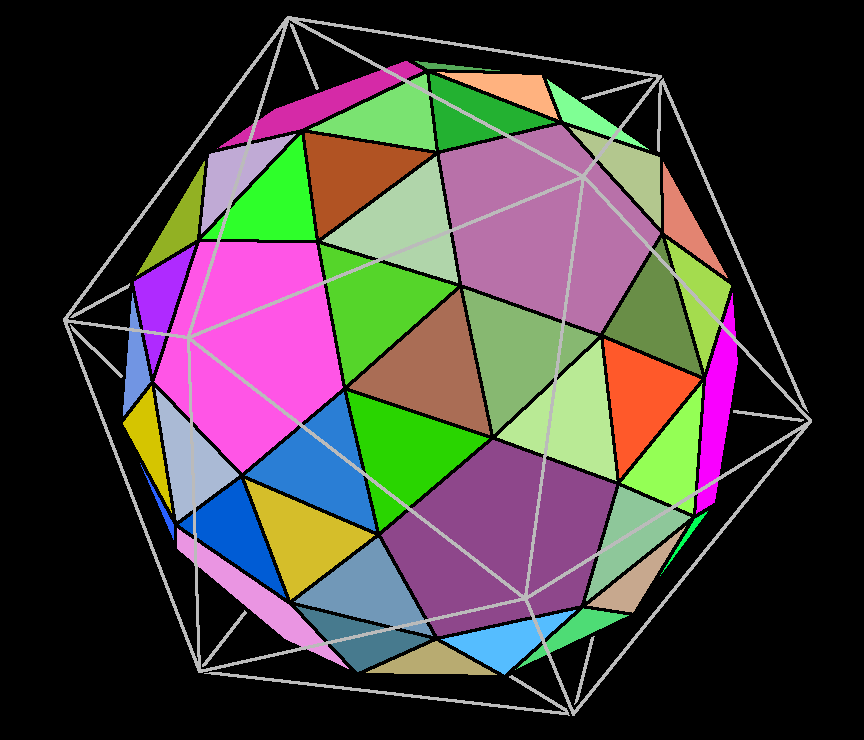Snub dodecahedron (aka snub icosidodecahedron). Snub truncation of icosahedron.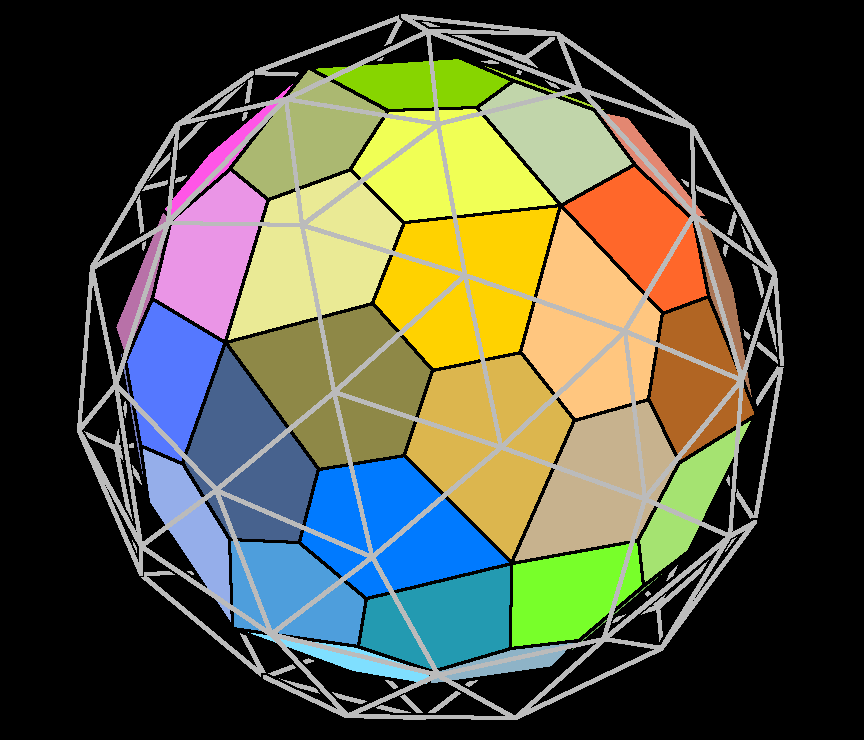Wireframe shows snub truncated icosahedron, inside is its dual, which has 60 congruent pentagon faces.

## Icosahedron Construction from Octahedron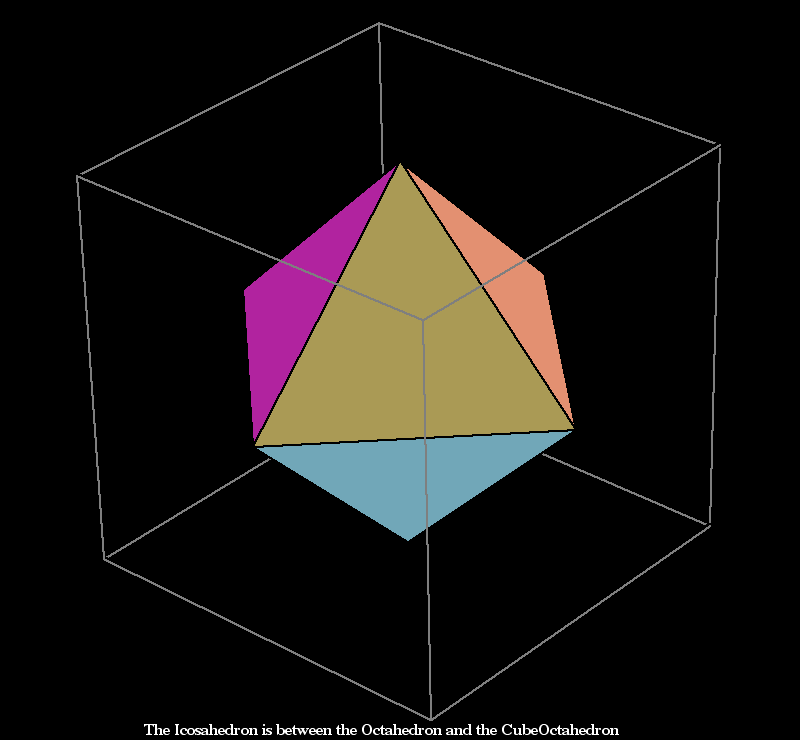Icosahedron Construction from Octahedron, by rotating a face of octahedron. When a newly created face becomes equilateral triangle, it is a icosahedron.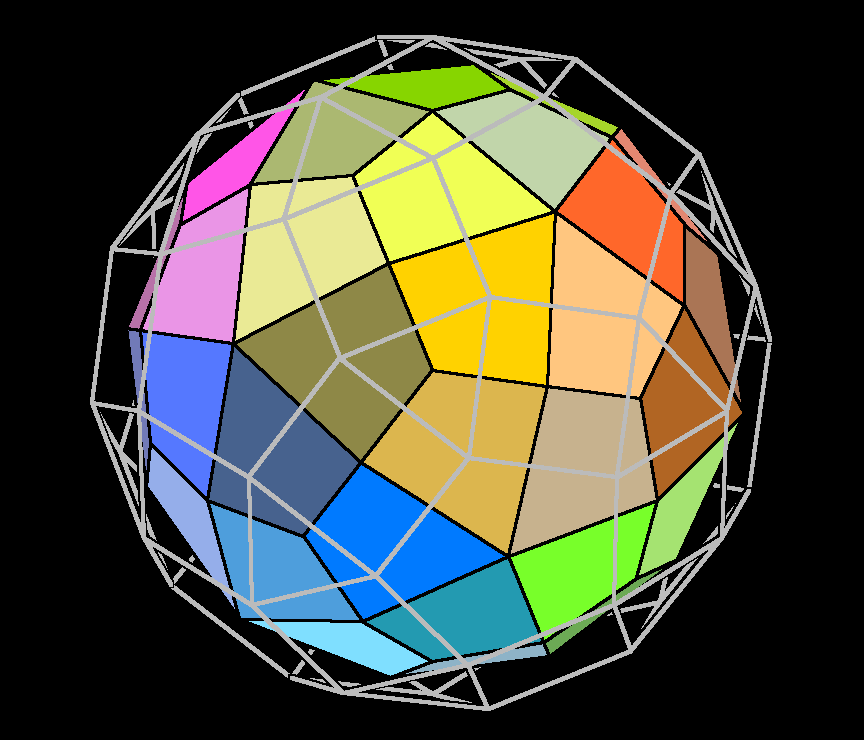Wireframe shows edge truncated icosahedron, inside is its dual, which has 60 congruent quadrilateral faces (kites).